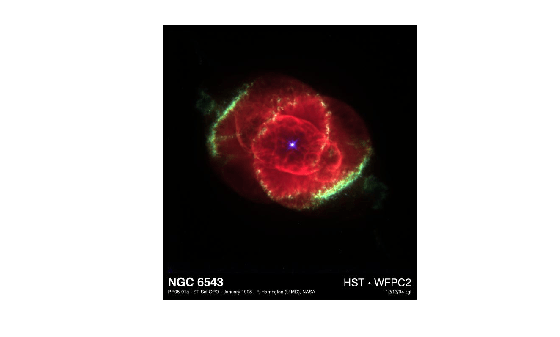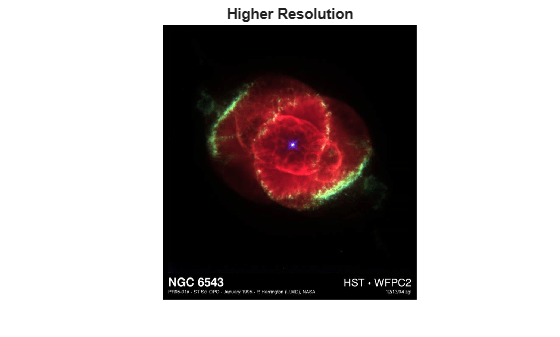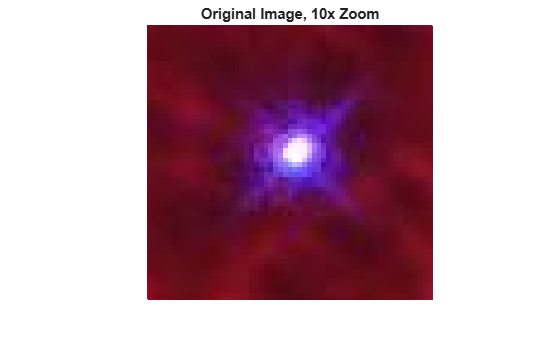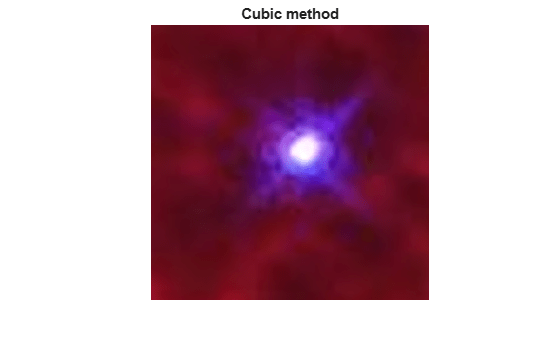# Resample Image with Gridded Interpolation

This example shows how to use `griddedInterpolant` to resample the pixels in an image. Resampling an image is useful for adjusting the resolution and size, and you also can use it to smooth out the pixels after zooming.

Load and show the image `ngc6543a.jpg`, which is a Hubble Space Telescope image of the planetary nebulae NGC 6543. This image displays several interesting structures, such as concentric gas shells, jets of high-speed gas, and unusual knots of gas. The matrix `A` that represents the image is a 650-by-600-by-3 matrix of uint8 integers.

```A = imread('ngc6543a.jpg'); imshow(A)```### Create Interpolant

Create a gridded interpolant object for the image. For images it is suitable to use the default grid, since the pixels have positive integer locations. Since `griddedInterpolant` only works for double-precision and single-precision matrices, convert the uint8 matrix to double precision.

`F = griddedInterpolant(double(A));`

### Resample Image Pixels

When you are resampling an image using a large number of grid points, the best way to query the interpolant is using grid vectors. The grid vectors are grouped together as column vectors in a cell array `{xg1,xg2,...,xgN}`. Grid vectors are a compact way to represent the grid of query points. With grid vectors, `griddedInterpolant` does not need to form the full grid to carry out the calculations.

Find the size of the original matrix dimensions, and use those dimension sizes to resample the image so that it is 120% the size. That is, for each 5 pixels in the original image, the interpolated image has 6 pixels.

```[sx,sy,sz] = size(A); xq = (0:5/6:sx)'; yq = (0:5/6:sy)'; zq = (1:sz)'; vq = uint8(F({xq,yq,zq})); figure imshow(vq) title('Higher Resolution')```Similarly, reduce the size of the image by querying the interpolant with 55% fewer points than the original image. While you can simply index into the original image matrix to produce lower resolution images, interpolation enables you to resample the image at noninteger pixel locations.

```xq = (0:1.55:sx)'; yq = (0:1.55:sy)'; zq = (1:sz)'; vq = uint8(F({xq,yq,zq})); figure imshow(vq) title('Lower Resolution')```### Smooth Out Zooming Artifacts

As you zoom in on an image, the pixels in the region of interest become larger and larger and detail in the image is quickly lost. You can use image resampling to smooth out these zooming artifacts.

Zoom in on the bright spot in the center of the original image. (The indexing into `A` is to center this bright spot in the image so that subsequent zooming does not push it out of the frame.)

```imshow(A(1:570,10:600,:),'InitialMagnification','fit') zoom(10) title('Original Image, 10x Zoom')```Query the interpolant `F` to reproduce this zoomed image (approximately) with 10x higher resolution. Compare the results from several different interpolation methods.

```xq = (1:0.1:sx)'; yq = (1:0.1:sy)'; zq = (1:sz)'; figure F.Method = 'linear'; vq = uint8(F({xq,yq,zq})); imshow(vq(1:5700,150:5900,:),'InitialMagnification','fit') zoom(10) title('Linear method')``````figure F.Method = 'cubic'; vq = uint8(F({xq,yq,zq})); imshow(vq(1:5700,150:5900,:),'InitialMagnification','fit') zoom(10) title('Cubic method')``````figure F.Method = 'spline'; vq = uint8(F({xq,yq,zq})); imshow(vq(1:5700,150:5900,:),'InitialMagnification','fit') zoom(10) title('Spline method')```